# Appendices¶

## Constants¶

List and meaning of the constants which are used for calculation.

• Conversion of geocentric coordinates into geodesic coordinates:
• ellipsoid semi-major axis: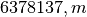;
• ellipsoid semi-minor axis: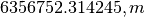.
• Calculation of elevation and azimuth:
• Earth’s radius: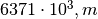.
• Calculation of geocentric coordinates of the GPS, GLONASS and GEO satellites:
• Earth’s angular velocity: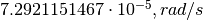;
• Earth’s gravitational field constant: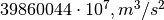;
• second zonal harmonic of geopotential expansion into a series of spherical functions: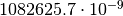;
• ellipsoid semi-major axis: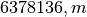(for GLONASS, according to PZ-90).

## Satellite system identifiers¶

The following is the list of the satellites system identifiers according to the RINEX format [RNX]:

• G - GPS
• R - GLONASS
• E - Galileo
• S - SBAS
• C - BeiDou